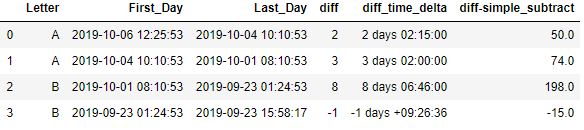# Working with Pandas datetime

In this post we will explore the Pandas datetime methods which can be used instantaneously to work with datetime in Pandas.

I am sharing the table of content in case you are just interested to see a specific topic then this would help you to jump directly over there

## Import time-series data

This is the monthly electrical consumption data in csv which we will import in a dataframe for this tutorial and this data can be downloaded using this link

### parse_dates attributes in read_csv() function

We are using `**parse_date**` attribute to parse and convert the date columns in the csv files to numpy datetime64 type

``````import pandas as pd
import numpy as np

df.info()
``````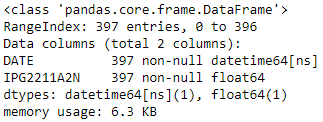### Pandas to_datetime

Alternatively, you can use to_datetime to convert any column to datetime

``````df['DATE']=pd.to_datetime(df['DATE'])
``````

## Extract Month and Year from datetime using datetime accessor

We will create 3 new columns here for Year, Month and day after extracting it from the Date column

``````df['Year']=df['DATE'].dt.year
df['month']=df['DATE'].dt.month
df['day']=df['DATE'].dt.day

# OR

df['Year']=df['DATE'].apply(lambda x: x.year)
df['month']=df['DATE'].apply(lambda x: x.month)
df['day']=df['DATE'].apply(lambda x: x.day)
``````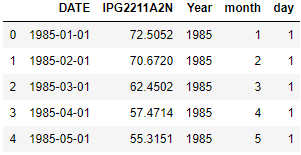## Time Series- Aggregation

### Resample to find sum on the date index date

resample() is a method in pandas that can be used to summarize data by date or time

Before re-sampling ensure that the index is set to datetime index i.e. DATE column here

Let’s find the Yearly sum of Electricity Consumption

``````df.set_index('DATE').resample('1Y').sum().head()
``````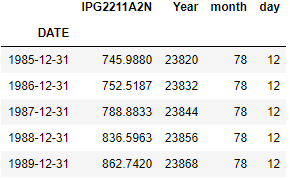### Resample to find mean on the date index date

Lets find the Electricity consumption mean for each year

``````df.set_index('DATE').resample('1Y').mean().head()
``````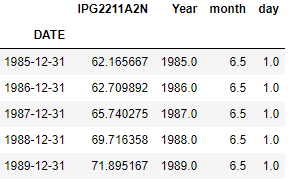## Datetime index and slice

Just ensure that the datetime column is set as index for the dataframe. I am using set_index() function to set that before index and slice

### Filter using the date

Get all the rows for year 1987

``````df.set_index('DATE')['1987'].head(2)
``````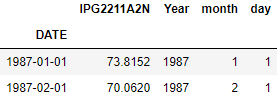### Filter all rows between two dates i.e. 1989-JAN and 1995-Apr here

Get all rows between JAN-1989 and APR-1995

``````df.set_index('DATE')['1989-01':'1995-04'].head()
``````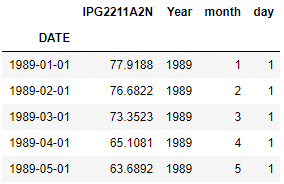## Date Offset

Its a kind of date increment used for a date range.

As per the documentation: Each offset specify a set of dates that conform to the DateOffset.

For example, Bday defines this set to be the set of dates that are weekdays (M-F). To test if a date is in the set of a DateOffset dateOffset we can use the onOffset method: dateOffset.onOffset(date).

If a date is not on a valid date, the rollback and rollforward methods can be used to roll the date to the nearest valid date before/after the date

DateOffsets can be created to move dates forward a given number of valid dates.

For example, Bday(2) can be added to a date to move it two business days forward. If the date does not start on a valid date, first it is moved to a valid date

### Add a day to DATE Column

Here we are adding a day(timedelta of 1 day) to the Date column in dataframe and creating a new column called as next_day

``````df['next_day']=df['DATE']+pd.Timedelta('1 day')
``````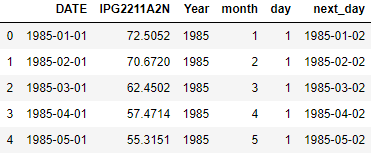Here we are adding a Business day using `Bday` param, it will add a day between Mon-Fri.

if a date is Sat then adding a `Bday` will return the next Monday i.e. a Business day instead of a Saturday

``````df['next_day']=df['DATE'].apply(lambda x: x+pd.offsets.BDay(1))
``````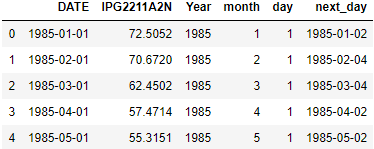Adding two days to the current DATE column using `days` parameter and create a new column day_after

``````df['day_after']=df['DATE'].apply(lambda x: x+pd.DateOffset(days=2))
``````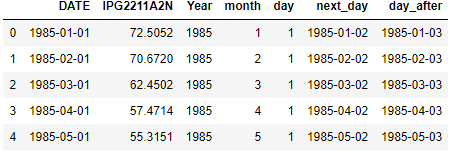Adding a month to the DATE column using `months` parameter

``````df['next_month_day']=df['DATE'].apply(lambda x: x+pd.DateOffset(months=1))
``````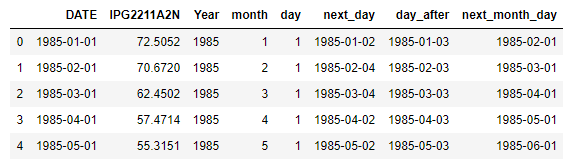For the complete list of parameters check this link

## Using date_range to create datetime index

it is Immutable numpy ndarray of datetime64 data.

We will see how to create datetime index and eventually create a dataframe using these datetime index arrays

### Datetime index with Hourly frequency

It gives the array of date and time starting from ‘2018-01-01’ with a Hourly frequency and period=3 means total elements of 3

``````import pandas as pd
dti = pd.date_range('2018-01-01', periods=3, freq='H')
dti
``````

DatetimeIndex([‘2018-01-01 00:00:00’, ‘2018-01-01 01:00:00’, ‘2018-01-01 02:00:00’], dtype=’datetime64[ns]’, freq=’H’)

### Monthly Frequency

Now change the frequency to Monthly and create array of total 10 dates

``````index = pd.date_range('2018-01-01',periods=10, freq='M')
index
``````
``````> _DatetimeIndex(['2018-01-31', '2018-02-28', '2018-03-31', '2018-04-30', '2018-05-31', '2018-06-30', '2018-07-31', '2018-08-31', '2018-09-30', '2018-10-31'],dtype='datetime64[ns]', freq='M')_
``````

### Weekly Frequency with start and end

Change the frequency to Weekly and create dates between two dates using start and end dates

``````pd.date_range(start='2019-01-01', end='2019-04-30', freq='W')
``````
``````> _DatetimeIndex(['2019-01-06', '2019-01-13', '2019-01-20', '2019-01-27', '2019-02-03', '2019-02-10', '2019-02-17', '2019-02-24', '2019-03-03', '2019-03-10', '2019-03-17', '2019-03-24', '2019-03-31', '2019-04-07', '2019-04-14', '2019-04-21', '2019-04-28'], dtype='datetime64[ns]', freq='W-SUN')_
``````

### Datetime index with start and end

``````import datetime
start = datetime.datetime(2011, 1, 1)

end = datetime.datetime(2011, 2, 1)

index = pd.date_range(start, end)
index
``````
``````> _DatetimeIndex(['2011-01-01', '2011-01-02', '2011-01-03', '2011-01-04', '2011-01-05', '2011-01-06', '2011-01-07', '2011-01-08','2011-01-09', '2011-01-10', '2011-01-11', '2011-01-12','2011-01-13', '2011-01-14', '2011-01-15', '2011-01-16','2011-01-17', '2011-01-18', '2011-01-19', '2011-01-20','2011-01-21', '2011-01-22', '2011-01-23', '2011-01-24', '2011-01-25', '2011-01-26', '2011-01-27', '2011-01-28', '2011-01-29', '2011-01-30', '2011-01-31', '2011-02-01'], dtype='datetime64[ns]', freq='D')_
``````

### Create dataframe using date time index

#### Create dataframe with datetime as index

Here index: dti is the date_range created above with hourly frequency

``````import numpy as np
df= pd.DataFrame({'price':np.random.uniform(0,20,size=3)},index=dti)
df
``````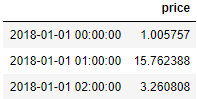#### Create dataframe with datetime as a column

``````import numpy as np
df= pd.DataFrame({'price':np.random.uniform(0,20,size=3),'date':dti})
df
``````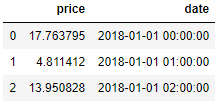### Datetime Index Using Holiday Calendar

You can also use the Holiday calendars to provide the list of holidays. Here we are using freq as US holiday calendar.

So the final datetime index will skip all the dates available in that holiday calendar

``````from pandas.tseries.holiday import USFederalHolidayCalendar

print (pd.date_range(start='2019-12-24',end='2019-12-31', freq=us_bd))
``````
``````> _DatetimeIndex(['2019-12-24', '2019-12-26', '2019-12-27', '2019-12-30', '2019-12-31'], dtype='datetime64[ns]', freq='C')_
``````

### Datetime Index using Origin Parameter

You can set the origin date and a list of days as a parameter and add that to origin date.

Here the origin is 2019-10-25 and adding 1 day to it gives 2019-10-26 and similarly adding 2 and 3 gives 2019-10-27 and 2019-10-28 resp

``````pd.to_datetime([1, 2, 3], unit='D', origin=pd.Timestamp('2019-10-25'))
``````
``````> _DatetimeIndex(['2019-10-26', '2019-10-27', '2019-10-28'], dtype='datetime64[ns]', freq=None)_
``````

One of the important feature is Week masking, In Middle eastern countries the working days in a week is thru Sun-Thu and Fri,Sat is considered as Weekends.

So here we are creating a dateindex using such working weeks from Sun-Thu and list of Holidays

``````weekmask = 'Sun Mon Tue Wed Thu'

holidays = [datetime.datetime(2011, 1, 5), datetime.datetime(2011, 3, 14)]

``````
``````> _DatetimeIndex(['2011-01-02', '2011-01-03', '2011-01-04', '2011-01-06', '2011-01-09', '2011-01-10', '2011-01-11', '2011-01-12','2011-01-13', '2011-01-16', '2011-01-17', '2011-01-18','2011-01-19', '2011-01-20', '2011-01-23', '2011-01-24','2011-01-25', '2011-01-26', '2011-01-27', '2011-01-30','2011-01-31', '2011-02-01'], dtype='datetime64[ns]', freq='C')_
``````

Using CustomBusinessdays you can create the custom business day using the same example of Middle eastern countries as shown above.

This will work exactly the same way as Dateoffset Bday() explained above.

As shown in the example here, If we add 2 Middle eastern business day to 2013-04-30 then it will return 2013-05-05 since Wed(2013-05-01) is a Holiday and Fri and Sat is a weekend so the 2nd business day is Sunday i.e. 2013-05-05

``````import datetime
import numpy as np
import pandas as pd

weekmask_egypt = 'Sun Mon Tue Wed Thu'

holidays = ['2012-05-01',datetime.datetime(2013, 5, 1),np.datetime64('2014-05-01')]

# dt = datetime.datetime(2013, 4, 30)
dt = pd.Timestamp('2013-04-30 17:00')
dt+2*bday_egypt
``````

Timestamp(‘2013-05-05 17:00:00’)

### Using Truncate

Two date attributes after and before is used to filter the records

``````df.truncate(after='2019-10')
``````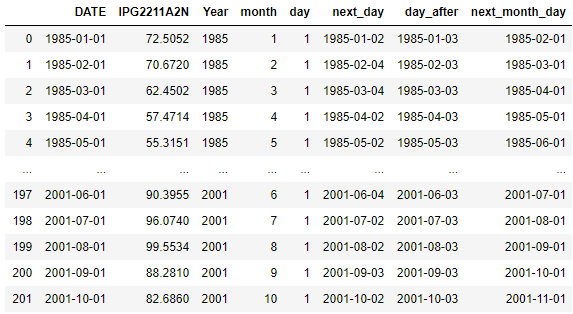## Timezones

Using parameter tz you can set the timezone for the timestamp, You can check the list of pytz timezones

``````ts = pd.Timestamp('2016-10-30 00:00:00', tz='Asia/Kolkata')
ts
``````

Timestamp(‘2016-10-30 00:00:00+0530’, tz=’Asia/Kolkata’)

### convert the timezone of a timestamp

Convert the timestamp to another timezone using tz_convert

``````pd.Timestamp('2016-10-30 00:00:00', tz='Asia/Kolkata').tz_convert('Europe/Amsterdam')
``````

Timestamp(‘2016-10-29 20:30:00+0200’, tz=’Europe/Amsterdam’)

``````bh = pd.offsets.BusinessHour()
pd.Timestamp('2016-10-30 00:00:00', tz='Asia/Kolkata')+2*bh
``````

Timestamp(‘2016-10-31 11:00:00+0530’, tz=’Asia/Kolkata’)

You can also set your own business hours with a start and end time

You can also set a CustomBusinessHours incorporating the Holiday Calendar list with a start and end business hours and weekmask as explained above

CustomBusinessHour(n=1, normalize=False, weekmask=’Mon Tue Wed Thu Fri’, holidays=None, calendar=None, start=’09:00’, end=’17:00’, offset=datetime.timedelta(0))

``````import datetime
from pandas.tseries.holiday import USFederalHolidayCalendar
dt = datetime.datetime(2014, 1, 17, 15)

dt+bhour_us
``````

Timestamp(‘2014-01-17 16:00:00’)

## Difference between two date columns

Lets see how to find difference between two datetime columns in dataframe in terms of no of days, seconds etc

``````import pandas as pd
from datetime import datetime
import numpy as np

# create dataframe
df = pd.DataFrame(data=[['A', '2019-10-06T12:25:53', '2019-10-04T10:10:53'],
['A', '2019-10-04T10:10:53', '2019-10-01T08:10:53'],
['B', '2019-10-01T08:10:53', '2019-09-23T01:24:53'],
['B', '2019-09-23T01:24:53', '2019-09-23T15:58:17']],
columns=['Letter', 'First_Day', 'Last_Day'])

df['First_Day']=pd.to_datetime(df['First_Day'])
df['Last_Day']=pd.to_datetime(df['Last_Day'])
df
``````

This is a dataframe with two datetime column i.e. First_Day and Last_Day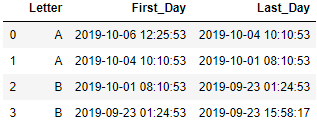### Difference between two dates in days and hours

diff column is created by subtracting the last_day and First_day which returns the difference in days

Similarly, diff_time_delta column returns the time-delta value

And finally the diff-simple_subtract column is difference in hours.

So for first row if you calculate the time-delta hours then it is 2 days and 2 hours which is equivalent to 50hrs which is the value in diff-simple_subtract

``````df['diff']=(pd.to_datetime(df['First_Day']) - pd.to_datetime(df['Last_Day'])).dt.days
df['diff_time_delta']=df['First_Day']-df['Last_Day']
df['diff-simple_subtract']=((df['First_Day']-df['Last_Day']).dt.total_seconds())//3600